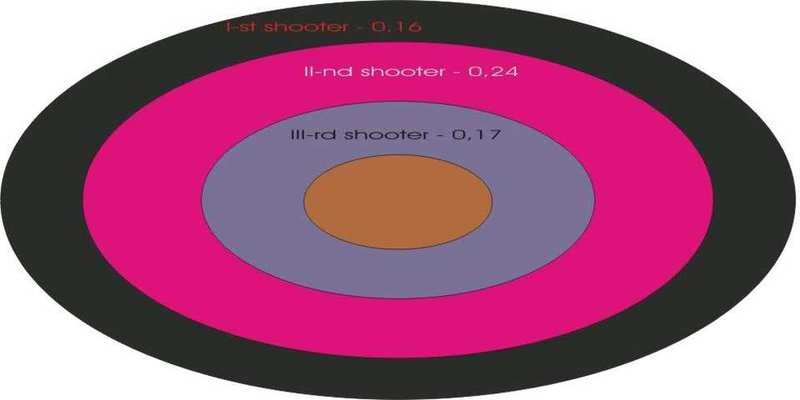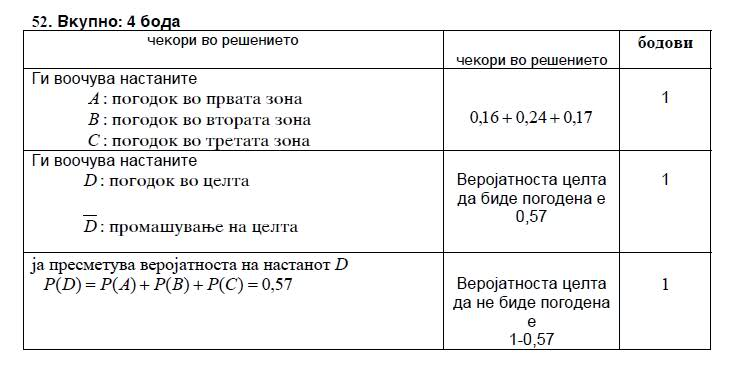# Matura exam results wrong ? (probabiltity of shooting the target)

matura exam results wrong !? (probabiltity of shooting the target)

Hello!
I got one task that I seem to solved it correctly. But the matura exam results are different then my solution.

Here is the task:
There is one target which is divided on three concentric zones. Here is picture of it:So the whole target is not the center of the darts, but the whole surface of the darts.

The task is to find the probability that the target will be missed by all of the shooters.

I find it like:

A - the Ist shooter hits the target

B - the IInd shooter hits the target

C- the IIIrd shooter hits the target

$$P(\bar{A} \cap \bar{B} \cap \bar{C}) = P(\bar{A})*P(\bar{B})*P(\bar{C})=(1-0,16)*(1-0,24)*(1-0,17)$$

And their solution is 1- P(A U B U C) which they wrote it like 1 - (P(A)+P(B)+P(C)).

Which solution is correct?

Mine or theirs?

Thanks in advance.

## Answers and Replies

HallsofIvy
Science Advisor
Homework Helper

I find the picture very confusing. It is divided into three areas and each area has a different probability but apparently those probabilities are the probabilities a specific shooter will hit the entire target. The three areas are irrelevant.
If P(A) is the probability that the first shooter will hit the target, P(B) the probability the second shooter will, and P(C) the probability the third shooter will hit the target, then what you give is correct.

What "they" give is incorrect. $1- (A\cup B\cup C)$ is the probability they do NOT all hit the target- the probability that at least one misses.
(And, by the way, $1- (A\cup B\cup C)$ is NOT 1- (P(A)+ P(B)+ P(C)). In fact, if P(A)+ P(B)+ P(C)> 1, which is quite plausible, that would give a negative probability! The probability of $1- (A\cup B\cup C)$ is 1- P(A)*P(B)*P(C).

I find the picture very confusing. It is divided into three areas and each area has a different probability but apparently those probabilities are the probabilities a specific shooter will hit the entire target. The three areas are irrelevant.
If P(A) is the probability that the first shooter will hit the target, P(B) the probability the second shooter will, and P(C) the probability the third shooter will hit the target, then what you give is correct.

What "they" give is incorrect. $1- (A\cup B\cup C)$ is the probability they do NOT all hit the target- the probability that at least one misses.
(And, by the way, $1- (A\cup B\cup C)$ is NOT 1- (P(A)+ P(B)+ P(C)). In fact, if P(A)+ P(B)+ P(C)> 1, which is quite plausible, that would give a negative probability! The probability of $1- (A\cup B\cup C)$ is 1- P(A)*P(B)*P(C).

Thanks for the reply HallsofIvy.

I also find the task very confusing. We have already discussed in other thread about this problem, and I already understood it.

I also realized that P(A)+ P(B)+ P(C) can be >1 and their solution is incorrect.

Here is their solution:D: the target is being hit

$$\bar{D}$$: the target is not being hit.

The other thing is all the same.

Thanks again for confirming.

Regards.